Next: 2.1.1 Neural networks for Up: 2. Modeling of data Previous: 2. Modeling of data

# 2.1 Principal component analysis (PCA)

Principal component analysis is a widely used tool for dimension reduction (Diamantaras and Kung, 1996). Let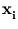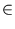IRd, where i = 1,..., n, be the training patterns. The principal components are a set of q < d orthonormal vectors and span a subspace in the major directions into which the patterns extend (figure 2.1).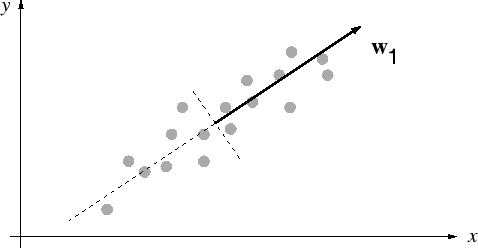In this section, we assume that the patterns are centered around the origin (without loss of generality). Let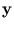be the projection onto a subspace,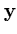=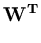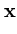. (2.1)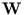is a d×q matrix that contains the principal components as columns. The vectoris a dimension-reduced representation of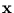. Let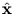be the reconstruction ofgiven only the vector,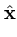=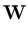. (2.2)

The goal of PCA is to set the subspace such that the mean reconstruction error Erec is minimized,

 Erec =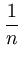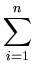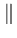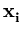-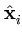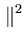. (2.3)

This goal is equivalent to finding the q major directions of maximal variance within the set of patterns {} (Diamantaras and Kung, 1996). Moreover, it is equivalent to the principal components being the first q eigenvectors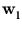of the covariance matrix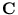of the pattern set (Diamantaras and Kung, 1996),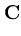=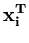. (2.4)

The corresponding eigenvalue equation is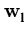=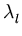. (2.5)

The eigenvalue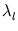is the variance of the distribution {} in the direction of. The following sections describe how neural networks can extract principal components and how PCA can be linked to the probability density of a pattern distribution.

SubsectionsNext: 2.1.1 Neural networks for Up: 2. Modeling of data Previous: 2. Modeling of data
Heiko Hoffmann
2005-03-22